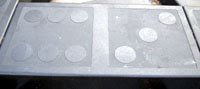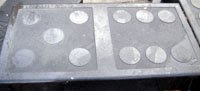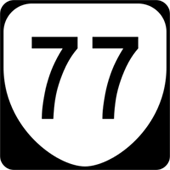## Wednesday, November 26, 2008

### 692

692 = 22 x 173.

692 is a number that has no digits in common with its cube (331373888).

692 has a representation as a sum of two squares: 692 = 42 + 162.

692 is a member of the sequence generated when you start with 1, then repeatedly multiply by 5 and reverse the digits: 1, 5, 52, 62, 13, . . . , 592, 692, 643, . . .In Microsoft Windows, error 692 signals a "hardware failure in port or attached device."

The U.S.S. Allen M. Sumner (DD-692), a destroyer, served in the U.S. Navy from 1943 to 1973.

## Tuesday, November 25, 2008

### 1225

1225 is the product of the square of 5 and the square of 7 and hence is the square of 35. It is the product of the squares of two consecutive primes, 5 and 7.

1225 has a representation as a sum of two squares: 1225 = 212 + 282.

1225 is the smallest number with three representations as a sum of four positive cubes: 1225 = 13 + 23 + 63 + 103 = 33 + 73 + 73 + 83 = 43 + 63 + 63 + 93.

1225 is a triangular square number; it is both a perfect square and a triangular number. 1225 is also a hexagonal number.A representation of consecutive hexagonal numbers.Pere Marquette 1225 is a steam locomotive.

## Monday, November 24, 2008

### 105

"Every number is built by multiplying prime numbers—105, for example, is three times five times seven. They are like the atoms of arithmetic—the hydrogen and oxygen of the world of numbers."—Marcus du Sautoy, The Guardian, Nov. 1, 2008.

105 is the 14th triangular number. It is the sum of consecutive integers: 1 + 2 + 3 + . . . + 13 + 14 = 105.

105 is the sum of consecutive integers in four other ways: 12 + 13 + . . . + 17 + 18 = 15 + 16 + 17 + 18 + 19 + 20 = 19 + 20 + 21 + 22 + 23 = 34 + 35 + 36 = 52 + 53.

Paul Erdös conjectured that
105 is the largest number n such that the positive values of n - 2k are all prime: 105 - 2 = 103, 105 - 4 = 101, 105 - 8 = 97; 105 - 16 = 89; 105 - 32 = 73; 105 - 64 = 41.

105 is 77 in base 14.Space Shuttle Mission STS-105 was launched into space on Aug. 10, 2001.

## Friday, November 21, 2008

### 2929

2929 = 29 x 101.

2929 has two representations as a sum of two squares: 2929 = 152 + 522 = 252 + 482.

2929 is the hypotenuse of two primitive Pythagorean triples: 29292 = 15602 + 24792 = 16792 + 24002.

2929 is a square-free number because its prime decomposition contains no repeated factors.

2929 is a divisor of 10014 - 1.2929 Entertainment is a media company with interests ranging from movie production to video games.

## Thursday, November 20, 2008

### 1875

1875 = 3 x 54

1875 is an economical number.

1875 is the smallest order for which there are 21 groups.

The product of the prime factors of 1875 plus one is a perfect square: 3 x 5 + 1 = 16 = 42.

1875 is a number n such that n - 4, n - 2, n + 2, and n + 4 are prime: 1871, 1873, 1877, and 1879 are all prime.French mathematician
Henri Lebesgue was born June 28, 1875.

## Wednesday, November 19, 2008

### 416

416 = 25 x 13.

416 is the number of subsets of the 15th roots of unity that add up to a real number.

416 has a representation as a sum of two squares: 416 = 42 + 202.

416 is a number with exactly 12 divisors: 1, 2, 4, 8, 13, 16, 26, 32, 52, 104, 208, 416.416 is the area code for the city of Toronto. It is one of the original 86 area codes from 1947.

Episode 416 (4th season, 16th episode) of the TV series Numb3rs was titled "Atomic No. 33" and included references to non-Newtonian fluids, the Riemann zeta function, and Bayesian network analysis.

## Tuesday, November 18, 2008

### 63

63 = 32 x 7.

63 = 62 + 33 = 26 – 1.

63 is the smallest number such that the common alphabetical value of its representation in Roman numerals is equal to itself: LXIII -- 12 + 24 + 9 + 9 + 9 = 63.

63 is a Harshad (or Niven) number; that is, it is divisible by the sum of its digits.

63 is the number of partially ordered sets of 5 elements.

63 is 333 in base 4.M63 is a galaxy, known as the Sunflower Galaxy, in the constellation Canes Venatici.

## Monday, November 17, 2008

### 145

145 = 5 x 29.

145 = 1! + 4! + 5! The only other numbers that are the sum of the factorials of their digits are 1, 2, and 40,585. These numbers are known as factorions.

145 is a pentagonal number and a centered square number.

145 is the sum of two squares in two different ways: 145 = 122 + 12 = 82 + 92.

145 is the hypotenuse of two primitive Pythagorean triples: 1452 = 172 + 1442 = 242 + 1432.Ted Williams hit 31 home runs and had 145 RBI as a rookie in 1939.

## Friday, November 14, 2008

### 118

118 = 2 x 59.

118 is the smallest number that can be written as the sum of four triples, whose products are all equal: 118 = 14 + 50 + 54 = 15 + 40 + 63 = 18 + 30 + 70 = 21 + 25 + 72. The product of each triple is 37,800.

118 is the smallest three-digit composite number, all of whose other digit permutations are prime numbers: 181 and 811 are both prime.

118 is the sum of three positive cubes: 118 = 43 + 33 + 33.118 Peitho is an asteroid that was discovered in 1872.

## Thursday, November 13, 2008

### 74

74 = 37 x 2.

74 = 17 + 18 + 19 + 20.

74 is a hungry number. The kth hungry number is the smallest number n such that 2n contains the first k digits of the decimal expansion of pi: 5, 17, 74, 144, 144, 2003, . . . . The first number is 5 because 25 is 32, which contains 3, the first digit of pi. They were named hungry numbers because they try to eat as much "pi" as possible.

74 has a representation as a sum of two squares: 74 = 52 + 72.74 is the atomic number of tungsten.

Source: Number Gossip

## Wednesday, November 12, 2008

### 56

56 = 23 x 7.

56
is a pronic number because it is the product of two consecutive numbers: 7 x 8 = 56.

56 is the sum of the first six triangular numbers: 1 + 3 + .6 + 10 + 15 + 21 = 56. Hence, it is a tetrahedral number, the number of balls you can put in a triangular pyramid: 1, 4, 10, 20, 35, 56, 84, 120, . . .

56 is the sum of six consecutive prime numbers: 3 + 5 + 7 + 11 + 13 + 17 = 56.56 men signed the Declaration of Independence in 1776, which led to the formation of the United States of America.

Source: Number Gossip

## Tuesday, November 11, 2008

### 1111

1111 = 11 x 101.

1111 is the sum of the digits of the first 100 primes.

1111 is a number that cannot be written as a sum of three squares.

1111 is a divisor of 1002 - 1.

1111 is n3 + n2 + n + 1, for n = 10.

1111 is 787 in base 12.Armistice Day, Nov. 11 (11/11), is the anniversary of the symbolic end of World War I in 1918, when the leaders of several countries signed an agreement on the 11th hour of the 11th day of the 11th month to cease hostilities.

Source:
Number Gossip

## Monday, November 10, 2008

### 77

77 = 7 x 11.

77 is the number of integer partitions of 12.

77 is the 22nd discrete biprime and the first of the (7.q) family. Since both 7 and 11 are Gaussian primes, this means that 77 is a Blum integer.

77 is the sum of three squares: 77 = 42 + 52 + 62.

77 is the sum of the first eight prime numbers: 77 = 2 + 3 + 5 + 7 + 11 + 13 + 17 + 19.During World War II in Sweden at the border with Norway, 77 was used as a password, because the tricky pronunciation in Swedish made it easy to instantly discern whether the speaker was native Swedish, Norwegian, or German.

Source: Wikipedia

## Friday, November 7, 2008

### 508

508 = 127 x 22

508 is the sum for four consecutive prime numbers: 113 + 127 + 131 + 137 = 508. It is also the sum of two successive primes: 508 = 251 + 257.

508 is a number that cannot be written as a sum of three squares.

508 is a member of the sequence generated by starting with 1 and repeatedly reversing the digits and adding two to get the next term: 1, 3, 5, 7, 9, 11, 13, 33, . . . , 605, 508, 607, . . .508 is the area code for Worcester, Mass.

In the United States, section 508 of the Rehabilitation Act requires that Federal agencies give disabled employees and members of the public access to information that is comparable to the access available to others.

Sources: Wikipedia

## Thursday, November 6, 2008

### 85

85 = 5 x 17.

85 is a Smith number because the sum of its digits is equal to sum of the digits in its prime factorization: 8 + 5 = 13 = 5 + 1 + 7.

85 is a centered triangular number. Such a number can be represented by a dot in the center, with all other dots surrounding the center in successive triangular layers. The first few triangular numbers are: 1, 4, 10, 19, 31, 46, 64, 85, 109, . . .85 is the largest known n for which 12 + 22 + 32 + . . . + n2 = 1 + 2 + 3 + . . . + m has a solution.There are 85 ways to tie a tie, according to a book by Thomas Fink and Yong Mao.

## Wednesday, November 5, 2008

### 538

538 = 2 x 269.

538 has a representation as a sum of two squares: 538 = 32 + 232.

538 is the 10th open meandric number: 1, 1, 2, 3, 8, 14, 42, 81, 262, 538, 1828, . . .

538 is a number such that the sum of its odd digits equals the sum of its even digits: 5 + 3 = 8.The Electoral College consists of 538 popularly elected representatives who formally select the President and the Vice President of the United States.

Source: WolframAlpha

## Tuesday, November 4, 2008

### 435

435 = 3 x 5 x 29.

435 is a triangular number and a hexagonal number.

435 is the number of ordered partitions of 16 into distinct parts.

435 is the sum of seven positive fifth powers: 435 = 25 + 25 + 25 + 25 + 25 + 25 + 35.

## Monday, November 3, 2008

### 1103

1103 is a prime number. It is also a Sophie Germain prime. If both p and 2p + 1 are prime, then p is a Sophie Germain prime: 1103 and 2207 are both primes.

11032 = 1216609 and 30112 = 9066121.

1103 is the number of graphs with 9 vertices and 8 edges.

1103 is a number that cannot be written as a sum of three squares.

1103 is a prime whose reversal is a different prime: 3011.The Intel
1103 DRAM, introduced in 1970, was the first chip to store a significant amount of information.